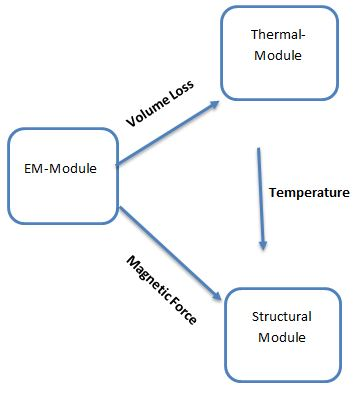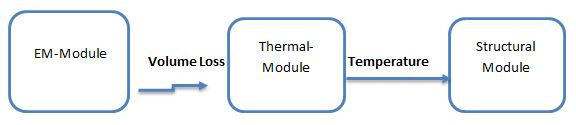# Structural Coupling

HOME / EMS / Structural Coupling

## Structural coupling

EMS comes also with an integrated linear static structural solver. EMS allows users to couple Electromagnetics with linear static stress. Electromagnetic phenomenon can apply forces to different parts then the structural coupling calculates the stress, the strain and the displacement due to these forces.

Deformation and thermal stress can also be computed and visualized through the Structural/Thermal coupling. Deformation can be caused by electromagnetic forces and temperature rise resulting from Joule heating, eddy current and core loss. All coupling is carried out in the same analysis study and on one same original model geometry and mesh. No export/import of data is required for the coupling. Non-electromagnetic loads and conditions can also be added to the coupling analysis in the form of mechanical force, moment, pressure, gravity, temperature, spring, tie constraint and rigid constraint.## Applications

The coupling to structural is used in evaluating the mechanical deformation caused by temperature and/or electromagnetic forces. It has many practical applications such as:

• MEMS
• Wire bonding
• Induction heating
• High voltage cables
• Busbars
• Permanent magnet halbach array
• DC linear actuators
• Coils

## Results

After running a study with the structural solution option, the following structural quantities can be visualized:

### Displacement

Ux : Displacement in x direction
Uy : Displacement in y direction
Uz : Displacement in z direction
Ur : The resultant displacement

### Stress

Von Mises Stress
SXX : Normal stress in the X direction
SYY : Normal stress in the Y direction
SZZ : Normal stress in the Z direction
SXY : Shear stress in the Y in the YZ plane
SYZ : Shear stress in the Z in the XZ plane
SZX : Shear stress in the Z in the YZ plane
PS1 : First principal stress
PS2 : Second principal stress
PS3 : Third principal stress
Stress intensity (P1-P3)

### Strain

Von Mises Strain
EXX : Normal strain in the X direction
EYY : Normal strain in the Y direction
EZZ : Normal strain in the Z direction
EXY : Shear strain  in the Y in the YZ plane
EYZ : Shear strain in the Z in the XZ plane
EZX : Shear strain in the Z in the YZ plane
PE1 : First principal strain
PE2 : Second principal strain
PE3 : Third principal strain
Tresca

### Reaction Force

RFX : Reaction Force in the X direction
RFY : Reaction Force in the Y direction
RFZ : Reaction Force in the Z direction

### 3D Safety Factor

Von Mises Stress Failure Criterion
Maximum shear stress Failure Criterion
Maximum Normal Stress Failure Criterion
Mohr Coulomb Failure Criterion

## Examples of design issues

The coupling to structural helps to study and solve many devices such as:

• Analyze the thermal stress in MEMS applications
• Evaluate the deformation caused by electric force in a micro actuator.
• Predict the stress caused by magnetic force in permanent magnet array.
• Study the failure caused by stress in different types of material
• Compute the deformation in coils due to the Lorentz force